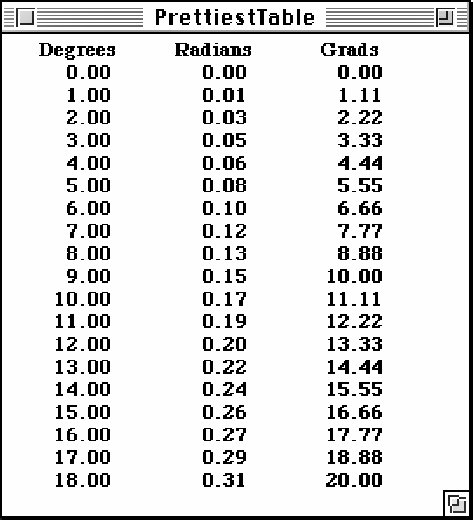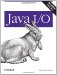# Specifying Width with FieldPosition

The Java core API does not include any classes that pad numbers with spaces like the traditional I/O APIs in Fortran, C, and other languages. Part of the reason is that its no longer a valid assumption that all output is written in a monospaced font on a VT-100 terminal. Therefore, spaces are insufficient to line up numbers in tables. Ideally, if you e writing tabular data in a GUI, you can use a real table component such as javax.swing.JTable. If thats not possible, you can measure the width of the string using a FontMetrics object and offset the position at which you draw the string. And if you are outputting to a terminal or a monospaced font, you can manually prefix the string with the right number of spaces.

The java.text.FieldPosition class separates strings into their component parts, called fields. (This is another unfortunate example of an overloaded term. These fields have nothing to do with the fields of a Java class.) For example, a typical date string can be separated into 18 fields including era, year, month, day, date, hour, minute, second, and so on. Of course, not all of these may be present in any given string. For example, 2006 CE includes only a year and an era field. The different fields that can be parsed are represented as public final static int fields (theres that annoying overloading again) in the corresponding format class. The java.text.DateFormat class defines these kinds of fields as mnemonic constants:

```public static final int ERA_FIELD
public static final int YEAR_FIELD
public static final int MONTH_FIELD
public static final int DATE_FIELD
public static final int HOUR_OF_DAY1_FIELD
public static final int HOUR_OF_DAY0_FIELD
public static final int MINUTE_FIELD
public static final int SECOND_FIELD
public static final int MILLISECOND_FIELD
public static final int DAY_OF_WEEK_FIELD
public static final int DAY_OF_YEAR_FIELD
public static final int DAY_OF_WEEK_IN_MONTH_FIELD
public static final int WEEK_OF_YEAR_FIELD
public static final int WEEK_OF_MONTH_FIELD
public static final int AM_PM_FIELD
public static final int HOUR1_FIELD
public static final int HOUR0_FIELD
public static final int TIMEZONE_FIELD
```

Number formats are a little simpler. They are divided into only two fields, the integer field and the fraction field. These are represented by the mnemonic constants NumberFormat.INTEGER_FIELD and NumberFormat.FRACTION_FIELD:

```public static final int INTEGER_FIELD
public static final int FRACTION_FIELD
```

The integer field is everything before the decimal point. The fraction field is everything after the decimal point. For instance, the string "-156.32" has an integer field of "-156" and a fraction field of "32".

The java.text.FieldPosition class identifies the boundaries of each field in the numeric string. You can then manually add the right number of monospaced characters or pixels to align the decimal points in a column of numbers. You create a FieldPosition object by passing one of these numeric constants into the FieldPosition( ) constructor:

```public FieldPosition(int field)
```

For example, to get the integer field:

```FieldPosition fp = new FieldPosition(NumberFormat.INTEGER_FIELD);
```

Theres a getField( ) method that returns this constant:

```public int getField( )
```

Next you pass this object into one of the format( ) methods that takes a FieldPosition object as an argument:

```NumberFormat nf = NumberFormat().getNumberInstance( );
StringBuffer sb = nf.format(2.71828, new StringBuffer( ), fp);
```

When format( ) returns, the FieldPosition object contains the start and end index of the field in the string. These methods return those items:

```public int getBeginIndex( )
public int getEndIndex( )
```

You can subtract getBeginIndex( ) from getEndIndex( ) to find the number of characters in the field. If you e working with a monospaced font, this may be all you need to know. If you e working with a proportionally spaced font, youll probably use java.awt.FontMetrics to measure the exact width of the field instead. Example 21-6 shows how to work in a monospaced font. This is essentially another version of the angle table. Now a FieldPosition object is used to figure out how many spaces to add to the front of the string; the getSpaces( ) method is simply used to build a string with a certain number of spaces.

##### Example 21-6. Prettier Table

 ```import java.text.*; public class PrettierTable { public static void main(String[] args) { NumberFormat myFormat = NumberFormat.getNumberInstance( ); FieldPosition fp = new FieldPosition(NumberFormat.INTEGER_FIELD); myFormat.setMaximumIntegerDigits(3); myFormat.setMaximumFractionDigits(2); myFormat.setMinimumFractionDigits(2); System.out.println("Degrees Radians Grads"); for (double degrees = 0.0; degrees < 360.0; degrees++) { String radianString = myFormat.format( radianString = getSpaces(3 - fp.getEndIndex( )) + radianString; String gradString = myFormat.format( gradString = getSpaces(3 - fp.getEndIndex( )) + gradString; String degreeString = myFormat.format( degrees, new StringBuffer(), fp).toString( ); degreeString = getSpaces(3 - fp.getEndIndex( )) + degreeString; System.out.println(degreeString + " " + radianString + " " + gradString); } } public static String getSpaces(int n) { StringBuffer sb = new StringBuffer(n); for (int i = 0; i < n; i++) sb.append( ); return sb.toString( ); } } ```

Heres some sample output. Notice the alignment of the decimal points:

```\$ java PrettierTable
0.00 0.00 0.00
1.00 0.02 1.11
2.00 0.03 2.22
3.00 0.05 3.33
4.00 0.07 4.44
5.00 0.09 5.56
6.00 0.10 6.67
7.00 0.12 7.78
8.00 0.14 8.89
9.00 0.16 10.00
10.00 0.17 11.11
11.00 0.19 12.22
12.00 0.21 13.33
13.00 0.23 14.44
```

This technique only works with monospaced fonts. In GUI environments, youll need to work with pixels instead of characters. Instead of prefixing a string with spaces, you adjust the position where the pen starts drawing each string. The getBeginIndex( ) and getEndIndex( ) methods, along with substring( ) in java.lang.String can be used to get the actual field, and the stringWidth( ) method in the java.awt.FontMetrics class can tell you how wide the field is.

Example 21-7 is yet another variant of the angle table. This one draws the angles in an applet. Figure 21-1 shows a screenshot of the running applet. This technique works equally well in a panel, frame, scroll pane, canvas, or other drawing environment with a paint( ) method.

##### Figure 21-1. The PrettiestTable applet##### Example 21-7. Prettiest Table applet

 ```import java.text.*; import java.applet.*; import java.awt.*; public class PrettiestTable extends Applet { NumberFormat myFormat = NumberFormat.getNumberInstance( ); FieldPosition fp = new FieldPosition(NumberFormat.INTEGER_FIELD); public void init( ) { this.setFont(new Font("Serif", Font.BOLD, 12)); myFormat.setMaximumIntegerDigits(3); myFormat.setMaximumFractionDigits(2); myFormat.setMinimumFractionDigits(2); } public void paint(Graphics g) { FontMetrics fm = this.getFontMetrics(this.getFont( )) ; int xmargin = 5; int lineHeight = fm.getMaxAscent() + fm.getMaxDescent( ); int y = lineHeight; int x = xmargin; int desiredPixelWidth = 3 * fm.getMaxAdvance( ); int fieldWidth = 6 * fm.getMaxAdvance( ); int headerWidth = fm.stringWidth("Degrees"); g.drawString("Degrees", x + (fieldWidth - headerWidth)/2, y); headerWidth = fm.stringWidth("Radians"); g.drawString("Radians", x + fieldWidth + (fieldWidth - headerWidth)/2, y); headerWidth = fm.stringWidth("Grads"); g.drawString("Grads", x + 2*fieldWidth + (fieldWidth - headerWidth)/2, y); for (double degrees = 0.0; degrees < 360.0; degrees++) { y += lineHeight; String degreeString = myFormat.format(degrees, new StringBuffer( ), fp).toString( ); String intPart = degreeString.substring(0, fp.getEndIndex( )); g.drawString(degreeString, xmargin + desiredPixelWidth - fm.stringWidth(intPart), y); String radianString = myFormat.format(Math.PI*degrees/180.0, new StringBuffer(), fp).toString( ); intPart = radianString.substring(0, fp.getEndIndex( )); g.drawString(radianString, xmargin + fieldWidth + desiredPixelWidth - fm.stringWidth(intPart), y); String gradString = myFormat.format(400 * degrees / 360, new StringBuffer(), fp).toString( ); intPart = gradString.substring(0, fp.getEndIndex( )); g.drawString(gradString, xmargin + 2*fieldWidth + desiredPixelWidth - fm.stringWidth(intPart), y); } } } ```Java I/O ISBN: 0596527500EAN: 2147483647 Year: 2004Pages: 244 Authors: Elliotte Rusty Harold BUY ON AMAZON Flylib.com © 2008-2020. If you may any questions please contact us: flylib@qtcs.net